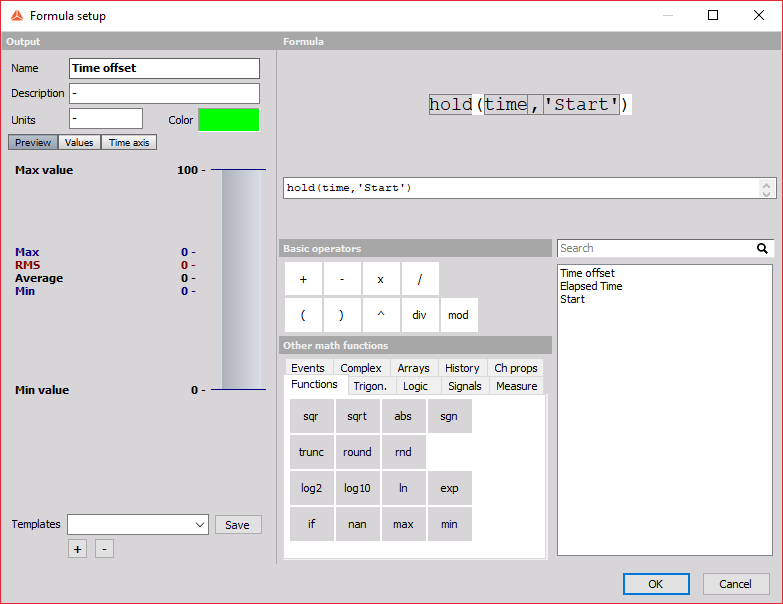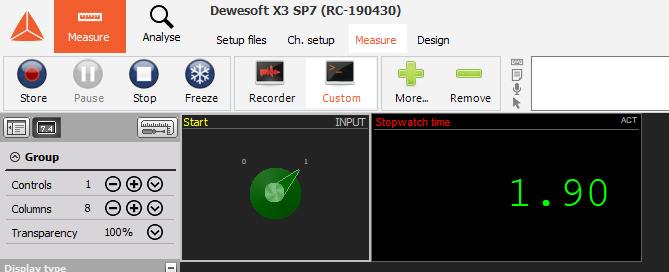A stopwatch comes in handy a lot of times during measurements. Here we will show you how to make a stopwatch which can be used in the measurement screen.

1. First we need to make a control channel called "Start".2. Now that we have our user input we have to make a time offset, which will store the time when we click on the "Start" button. We do this by using the "hold" function in Formula, which will hold a certain value at the point when a trigger condition appears. The value that we will hold is time and the trigger condition is the user input "Start" which will have a value of '1' when we press on the button in the measurement screen.3. Now we make a formula which subtracts the offset from the total time of the measurement.4. In the last formula we will make an if statement. Here we will increase the time only if the Start button is pressed and if it is not it will show the last value of the time.5. In the measurement screen we can now add an "input control display" and a "digital meter". On the input control we select the user input "Start" and we set it as a switch. On the digital meter we select the stopwatch time. If we turn the switch to '1' the time on digital meter will start running from 0 and if we turn the switch back to '0' the meter will display the elapsed time.